Classification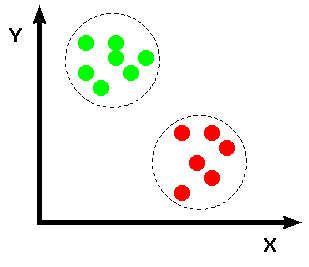Common Names: Classification

Brief Description

Classification includes a broad range of decision-theoretic approaches to the identification of images (or parts thereof). All classification algorithms are based on the assumption that the image in question depicts one or more features (e.g., geometric parts in the case of a manufacturing classification system, or spectral regions in the case of remote sensing, as shown in the examples below) and that each of these features belongs to one of several distinct and exclusive classes. The classes may be specified a priori by an analyst (as in supervised classification) or automatically clustered (i.e. as in unsupervised classification) into sets of prototype classes, where the analyst merely specifies the number of desired categories. (Classification and segmentation have closely related objectives, as the former is another form of component labeling that can result in segmentation of various features in a scene.)How It Works

Image classification analyzes the numerical properties of various image features and organizes data into categories. Classification algorithms typically employ two phases of processing: training and testing. In the initial training phase, characteristic properties of typical image features are isolated and, based on these, a unique description of each classification category, i.e. training class, is created. In the subsequent testing phase, these feature-space partitions are used to classify image features.
The description of training classes is an extremely important component of the classification process. In supervised classification, statistical processes (i.e. based on an a priori knowledge of probability distribution functions) or distribution-free processes can be used to extract class descriptors. Unsupervised classification relies on clustering algorithms to automatically segment the training data into prototype classes. In either case, the motivating criteria for constructing training classes is that they are:

• independent, i.e. a change in the description of one training class should not change the value of another,
• discriminatory, i.e. different image features should have significantly different descriptions, and
• reliable, all image features within a training group should share the common definitive descriptions of that group.

A convenient way of building a parametric description of this sort is via afeature vector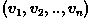, where n is the number of attributes which describe each image feature and training class. This representation allows us to consider each image feature as occupying a point, and each training class as occupying a sub-space (i.e. a representative point surrounded by some spread, or deviation), within the n-dimensional classification space. Viewed as such, the classification problem is that of determining to which sub-space class each feature vector belongs.
For example, consider an application where we must distinguish two different types of objects (e.g. bolts and sewing needles) based upon a set of two attribute classes (e.g. length along the major axis and head diameter). If we assume that we have a vision system capable of extracting these features from a set of training images, we can plot the result in the 2-D feature space, shown in Figure 1.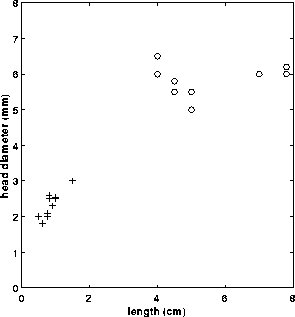Figure 1 Feature space: + sewing needles, o bolts.

At this point, we must decide how to numerically partition the feature space so that if we are given the feature vector of a test object, we can determine, quantitatively, to which of the two classes it belongs. One of the most simple (although not the most computationally efficient) techniques is to employ a supervised, distribution-free approach known as the minimum (mean) distance classifier. This technique is described below.Minimum (Mean) Distance Classifier
Suppose that each training class is represented by a prototype (or mean) vector: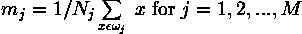where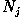is the number of training pattern vectors from class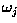. In the example classification problem given above,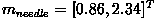and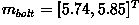as shown in Figure 2.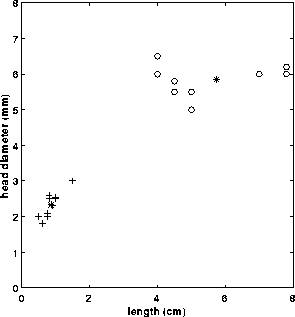Figure 2 Feature space: + sewing needles, o bolts, * class mean

Based on this, we can assign any given pattern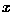to the class of its closest prototype by determining its proximity to each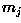. If Euclidean distance is our measure of proximity, then the distance to the prototype is given byIt is not difficult to show that this is equivalent to computing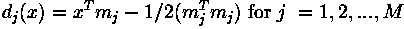and assignto classif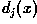yields the largest value.
Returning to our example, we can calculate the following decision functions: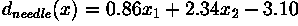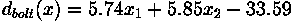Finally, the decision boundary which separates class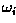fromis given by values forfor which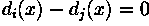In the case of the needles and bolts problem, the decision surface is given by: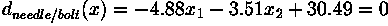As shown in Figure 3, the surface defined by this decision boundary is the perpendicular bisector of the line segment joining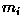and.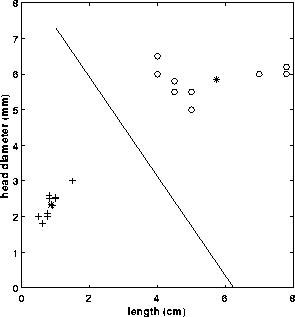Figure 3 Feature space: + sewing needles, o bolts, * class mean, line = decision surface

In practice, the minimum (mean) distance classifier works well when the distance between means is large compared to the spread (or randomness) of each class with respect to its mean. It is simple to implement and is guaranteed to give an error rate within a factor of two of the ideal error rate, obtainable with the statistical, supervisedBayes' classifier. The Bayes' classifier is a more informed algorithm as the frequencies of occurrence of the features of interest are used to aid the classification process. Without this information the minimum (mean) distance classifier can yield biased classifications. This can be best combatted by applying training patterns at the natural rates at which they arise in the raw training set.Guidelines for Use

To illustrate the utility of classification (using the minimum (mean) distance classifier), we will consider a remote sensing application. Here, we have a collection of multi-spectral images (i.e. images containing several bands, where each band represents a single electro-magnetic wavelength or frequency) of the planet Earth collected from a satellite. We wish to classify each image pixel into one of several different classes (e.g. water, city, wheat field, pine forest, cloud, etc.) on the basis of the spectral measurement of that pixel.
Consider a set of images of the globe (centered on America) which describe the visible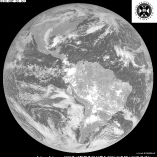and infra-red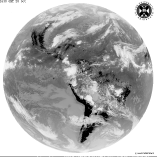spectrums, respectively. From the histograms of the visible band image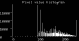and infra-red band image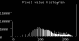we can see that it would be very difficult to find a threshold, or decision surface, with which to segment the images into training classes (e.g. spectral classes which correspond to physical phenomena such as cloud, ground, water, etc.). It is often the case that having a higher dimensionality representation of this information (i.e. using one 2-D histogram instead of two 1-D histograms) facilitates segmentation of regions which might overlap when projected onto a single axis, as shown for some hypothetical data in Figure 4.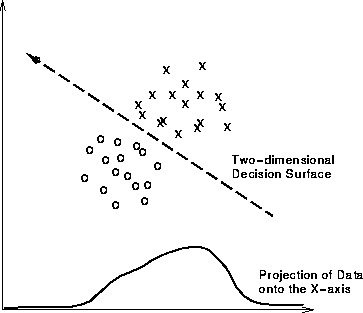Figure 4 2-D feature space representation of hypothetical data. (The projection of the data onto the X-axis is equivalent to a 1-D histogram.)

Since the images over America are registered, we can combine them into a single two-band image and find the decision surface(s) which divides the data into distinct classification regions in this higher dimensional representation. To this aim, we use ak-means algorithm to find the training classes of the 2-D spectral images. (This algorithm converts an input image into vectors of equal size (where the size of each vector is determined by the number of spectral bands in the input image) and then determines the k prototype mean vectors by minimizing of the sum of the squared distances from all points in a class to the class center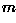.)
If we choose k=2 as a starting point, the algorithm finds two prototype mean vectors, shown with a * symbol in the 2-D histogram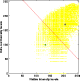This figure also shows the linear decision surface which separates out our training classes.
Using two training classes, such as those found for the image over America, we can classify a similar multi-spectral image of Africa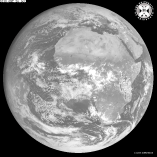(visible) and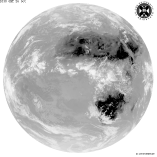(infra-red) to yield the result:(Note that the image size has been scaled by a factor of two to speed up computation, and a border has been placed around the image to mask out any background pixels.) We can see that one of the classes created during the training process contains pixels corresponding to land masses over north and south Africa, whereas the pixels in the other class represent water or clouds.
Classification accuracy using the minimum (mean) distance classifier improves as we increase the number of training classes. The images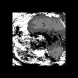and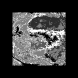show the results of the classification procedure using k=4 and k=6 training classes. The equivalent with a color assigned to each class is shown in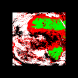and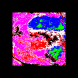for k=4 and k=6, respectively. Here we begin to see the classification segmenting out regions which correspond to distinct physical phenomena.

Common Variants

Classification is such a broad ranging field, that a description of all the algorithms could fill several volumes of text. We have already discussed a common supervised algorithm, therefore in this section we will briefly consider a representative unsupervised algorithm. In general, unsupervised clustering techniques are used less frequently, as the computation time required for the algorithm to learn a set of training classes is usually prohibitive. However, in applications where the features (and relationships between features) are not well understood, clustering algorithms can provide a viable means for partitioning a sample space.
A general clustering algorithm is based on asplit and merge technique, as shown in Figure 5. Using a similarity measure (e.g. the dot product of two vectors, the weighted Euclidean distance, etc.), the input vectors can be partitioned into subsets, each of which should be sufficiently distinct. Subsets which do not meet this criterion are merged. This procedure is repeated on all of the subsets until no further splitting of subsets occurs or until some stopping criteria is met.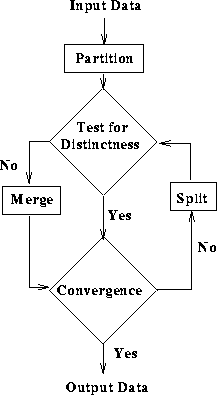Figure 5 General clustering algorithm

Exercises

1. In the classification of natural scenes, there is often the problem that features we want to classify occur at different scales. For example, in constructing a system to classify trees, we have to take into account that trees close to the camera will appear large and sharp, while those at some distance away may be small and fuzzy. Describe how one might overcome this problem.
2. The following table gives some training data to be used in the classification of flower types. Petal length and width are given for two different flowers. Plot this information on a graph (utilizing the same scale for the petal length and petal width axes) and then answer the questions below.

------------------------------------
| Petal Length | Petal Width | Class |
|--------------+-------------+-------|
| 4            | 3           | 1     |
|--------------+-------------+-------|
| 4.5          | 4           | 1     |
|--------------+-------------+-------|
| 3            | 4           | 1     |
|--------------+-------------+-------|
| 6            | 1           | 2     |
|--------------+-------------+-------|
| 7            | 1.5         | 2     |
|--------------+-------------+-------|
| 6.5          | 2           | 2     |
------------------------------------

a) Calculate the mean, or prototype, vectorsfor the two flower types described above. b) Determine the decision functions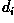for each class. c) Determine the equation of the boundary (i.e.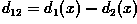) and plot the decision surface on your graph. d) Notice that substitution of a pattern from class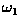into your answer from the previous section yields a positive valued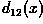, while a pattern belonging to the class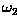yields a negative value. How would you use this information to determine a new pattern's class membership?
3. Experiment with classifying some remotely sensed images: e.g.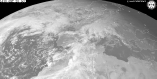and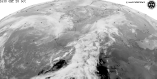are the visible and infra-red images of Europe,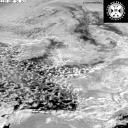and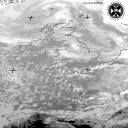are those of the United Kingdom and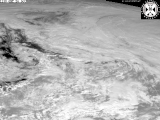and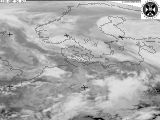are those of Scandinavia. Begin by combining the two single-band spectral images of Europe into a single multi-band image. (You may want to scale the image so as to cut down the processing time.) Then, create a set of training classes, where k equals 6,8,10... (Remember that although the accuracy of the classification improves with greater numbers of training classes, the computational requirements increase as well.) Then try classifying all three images using these training sets.

References

T. Avery and G. Berlin Fundamentals of Remote Sensing and Airphoto Interpretation, Maxwell Macmillan International, 1985, Chap. 15.
D. Ballard and C. Brown Computer Vision, Prentice-Hall, Inc., 1982, Chap. 6.
E. Davies Machine Vision: Theory, Algorithms and Practicalities, Academic Press, 1990, Chap. 18.
A. Jain Fundamentals of Digital Image Processing, Prentice-Hall, 1986, Chap. 9.
D. Vernon Machine Vision , Prentice-Hall, 1991, Chap. 6.